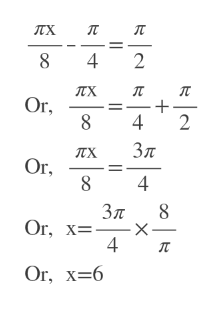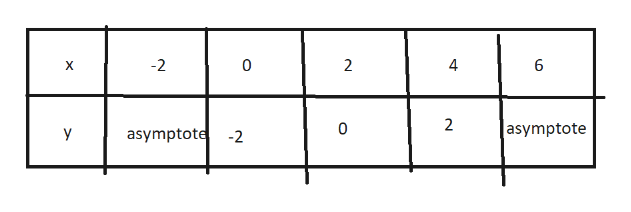1: consider y=2tan(pi x  -  pi)                               ___     ____                              8          4a)find the period of the above equation and sketch a full cycle of the graph including asymptotes, and appropriate scales.

Question

1: consider y=2tan(pi x  -  pi)

___     ____

8          4

a)find the period of the above equation and sketch a full cycle of the graph including asymptotes, and appropriate scales.

Step 1

Period does not depend on horizontal shift and vertical stretch.

Period= pi/b=pi/(pi/8)=8

Step 2

The graph has asymptotes when pi*x/8 - pi/4=pi/2

so, x=6

And at x=6-8=-2 there will be asymptote toohelp_outlineImage Transcriptioncloseлх п л 4 2 лх л л Or 8 4 2 Зл лх Or 8 4 Зл Or, х- 4 л Or, х-6 + fullscreen
Step 3

We draw from x=6-8=-2 to x=6, mak...help_outlineImage Transcriptionclose-2 0 2 4 6 X 2 |asymptote 0 asymptote Y -2 fullscreen

Want to see the full answer?

See Solution

Want to see this answer and more?

Our solutions are written by experts, many with advanced degrees, and available 24/7

See Solution
Tagged in

Other